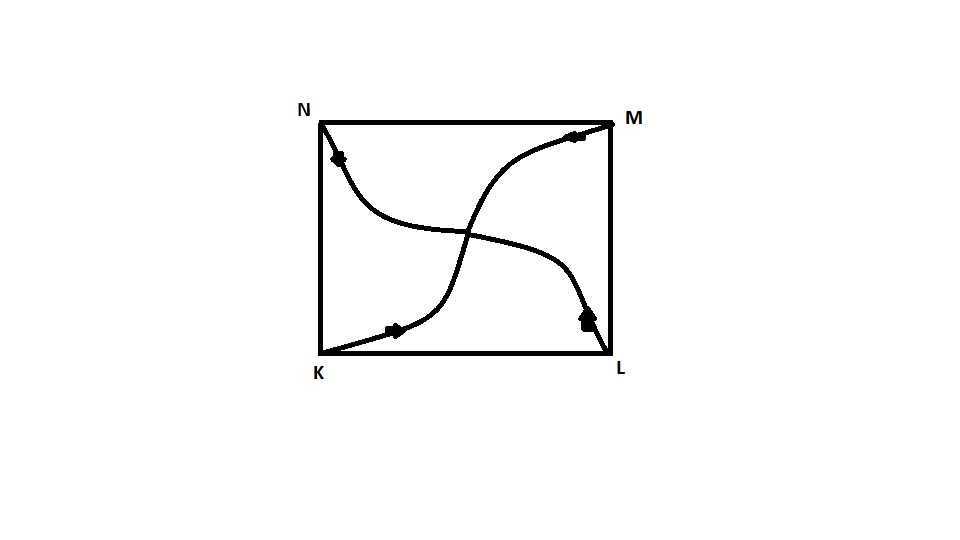# Four persons K, L, M, and N are initially at the four corners of a square of side d. Each persons now moves with a uniform speed v in such a way that K always moves directly towards L, L directly towards M, M directly towards N and N directly toward K. The four persons will meet at a time ..........??

9 years ago

Each of them will meet at centre of the square as shown in figure..  After every unit time , they will be forming a square..Let the centre be O. Now distance ON will be d/√2.

N is moving towards K and  angle KNO is 45 degree.
The component of velocity of K which will Cover the distance ON is" v cos 45= v/√2".

Now, time taken, t to cover the distance ON will be = (d/√2)/(v/√2)="d/v".     (Ans.)
Similarly, we can solve for any regular closed figure... like triangle, hexagon etc...

2 years ago
Dear Student,

Take reference from the image
Each person moves with constant speed towards the other but its velocity changes continuously.
They will meet at the center Finally.
After every Unit time they will from smaller square
Component of Velocity Towards center = v cos45
= v/√2​
Distance covered = (√(2d)/​​2) = ((diagonal)/2)​
= d/√2​
Time taken t = d/√2​/v/√2​
t = d/v​ AnsThanks and Regards